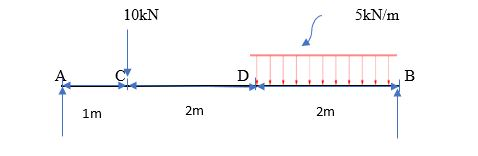10kN5kN/m1m2m2m

Question

Draw shear force and bending moment diagrams for the given simply supported beam.help_outlineImage Transcriptionclose10kN 5kN/m 1m 2m 2m fullscreen
Step 1

Draw a free body diagram converting the UDL into a point load

As UDL is given 5kn and it is acting on the 2m length

Therefore, load will be 5 x 2 = 10 kN acting on center of 1m from point B.

Step 2

Take moments @A, we know the condition

Anticlockwise moment = clockwise moments

We know moment = force x perpendicular distance

Rx 5 = (10 x 1) + (10 x 4)

Rx 5 = 50

RB = 50/5= 10 kN.

Summation of upward force = summation of downward force

R+ R= 10+10

RA + 10 = 20

R= 20 – 10

R= 10 kN

Step 3

Shear force calculations:

Shear force is the algebraic sum of unbalanced vertical forces to left or right of the section.

So, if we start calculating from left side then upward force will be positive and downward force will be negative and if we start calculating from the right side of the sect...

Want to see the full answer?

See Solution

Want to see this answer and more?

Our solutions are written by experts, many with advanced degrees, and available 24/7

See Solution
Tagged in

Civil Engineering